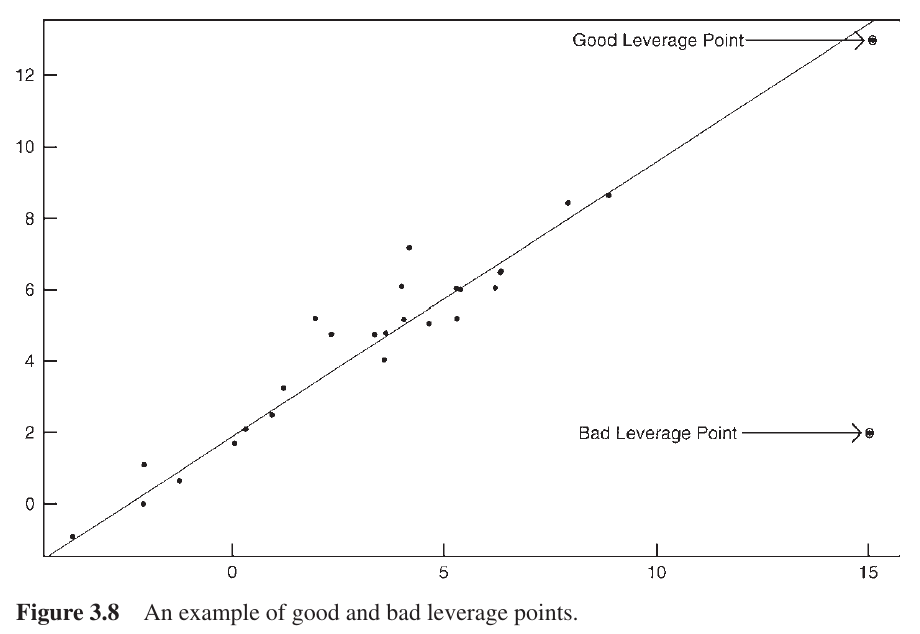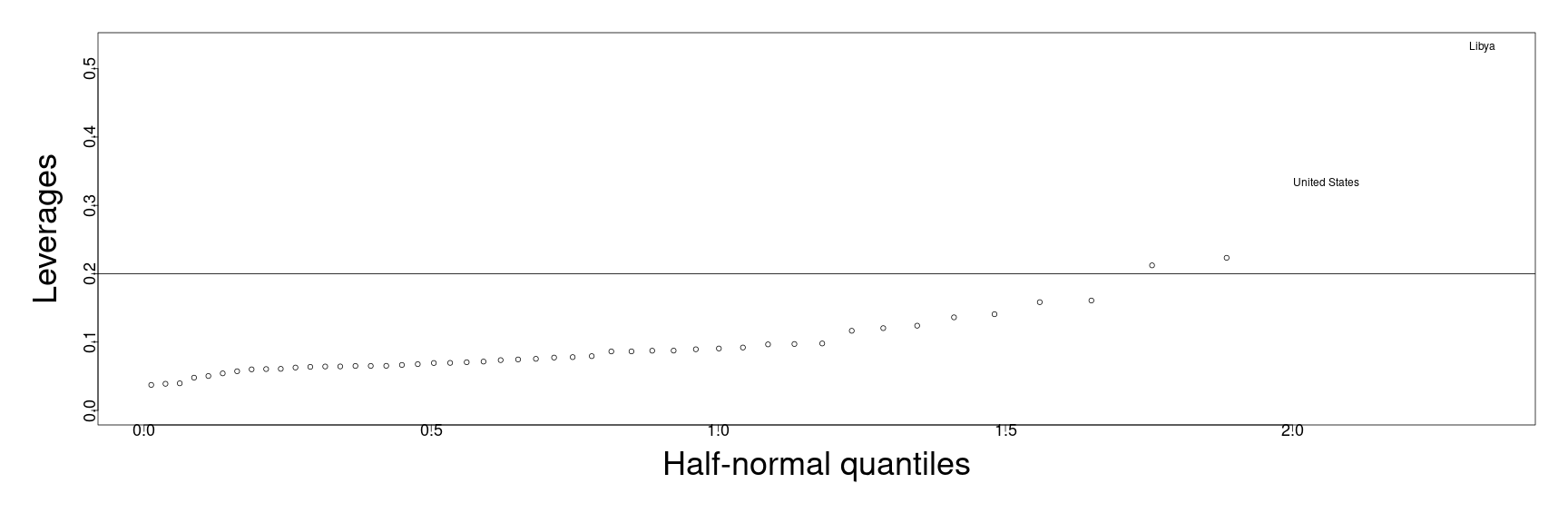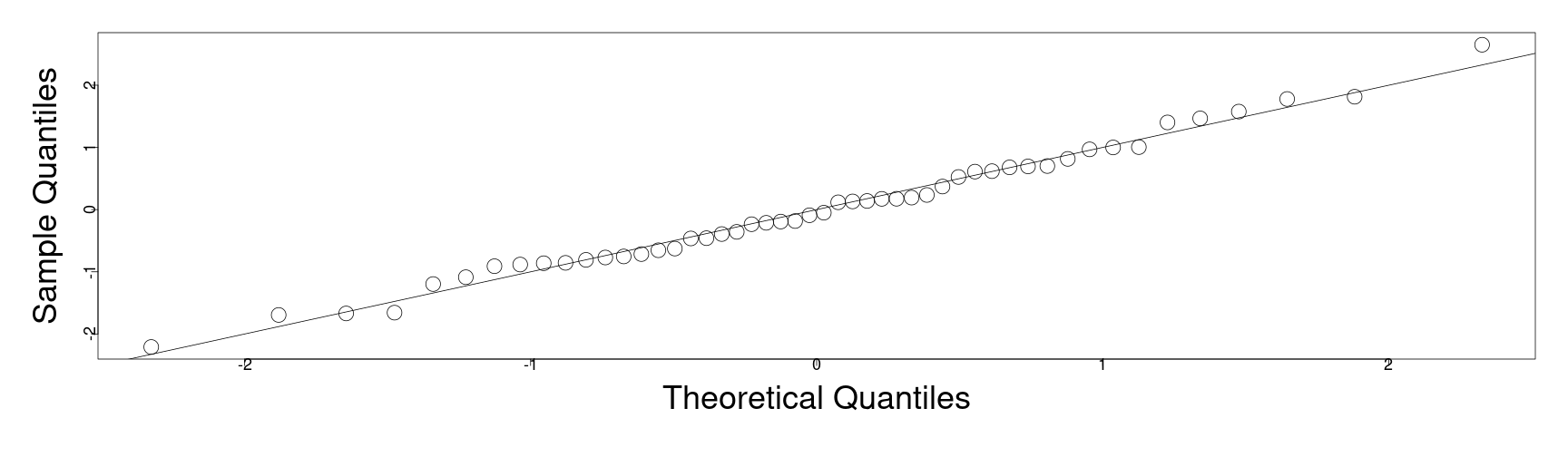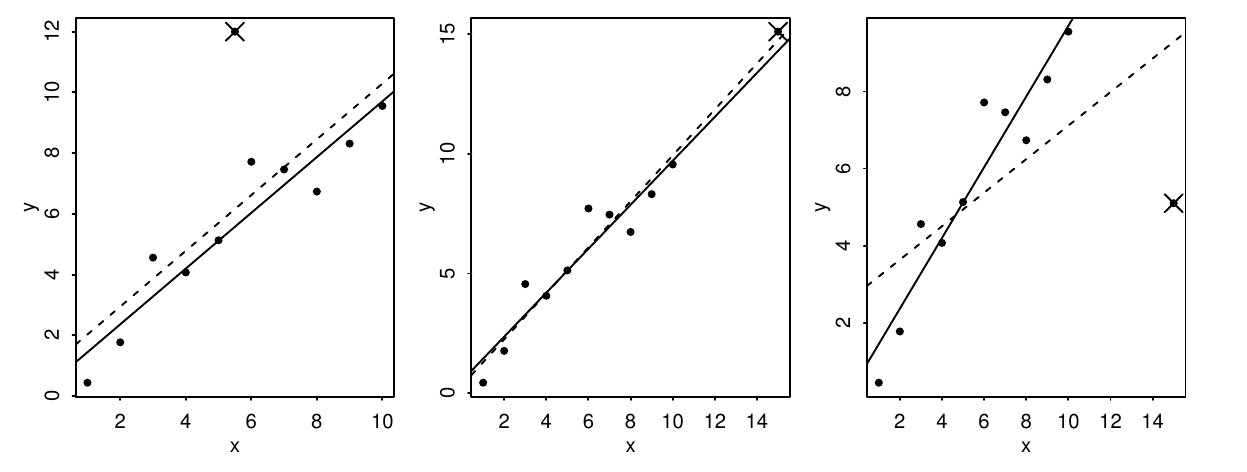# Diagnostics part 3: unusual observations

10/26/2020

## Instructions:

Use the left and right arrow keys to navigate the presentation forward and backward respectively. You can also use the arrows at the bottom right of the screen to navigate with a mouse.

FAIR USE ACT DISCLAIMER:
This site is for educational purposes only. This website may contain copyrighted material, the use of which has not been specifically authorized by the copyright holders. The material is made available on this website as a way to advance teaching, and copyright-protected materials are used to the extent necessary to make this class function in a distance learning environment. The Fair Use Copyright Disclaimer is under section 107 of the Copyright Act of 1976, allowance is made for “fair use” for purposes such as criticism, comment, news reporting, teaching, scholarship, education and research.

## Outline

• The following topics will be covered in this lecture:
• Leverage and diagnosing leverage
• Standardized residuals and outliers
• Bonferroni correction
• Influence and Cook's distance
• Parameter sensitivity
• Model plot function

## Diagnostics

• We are considering 4 categories of potential issues with the model:
1. Issues with the Gaussian error assumption: our hypothesis testing studied thus far relies on the Gaussian error assumption.
2. Issues with the form of the hypothetical covariance: we have assumed that $\mathrm{cov}\left(\mathbf{Y}\right) = \sigma^2\mathbf{I}$ but for many cases this will not be true.
3. Issues with unusual observations: some of the observations may not look like others, and they might change the choice and the fit of the model.
4. Issues with the systematic part of the model: we have assumed that there is an actual signal in the data of the form \begin{align} \mathbb{E}[\mathbf{Y}] = \mathbf{X} \boldsymbol{\beta}, \end{align} which may not be valid.

## Unusual observations

• We will now consider diagnostics for considering unusual observations.

• This will not refer to missing data, which will be treated near the end of the course, but rather data that is given and could change the interpretation of the statistical relationship.
• Some observations can have their own dramatic impact on the performance of the model,

• we denote these observations influential observations
• Outliers are those observations that don't fit the overall trend or the linear model well.

• It is possible that an observation is either, both or neither an outlier or influential.

• A leverage point is an extreme value of one of the explanatory variables, that has potential to influence the fit of the model.

• It is important to identify any of the above such points, but the choice of what to do with them depends strongly on the problem at hand.

### Leverage

• We will first study the leverage points.

• Recall the “hat” matrix that projects the observations into the space of the explanatory variables,

\begin{align} \mathbf{H}&\triangleq \mathbf{X}\left(\mathbf{X}^\mathrm{T}\mathbf{X} \right)^{-1}\mathbf{X}^\mathrm{T} \end{align}

• Using the hat matrix, we define the fitted values via the relationship,

\begin{align} \hat{\mathbf{Y}} = \mathbf{H}\mathbf{Y}. \end{align}

• Therefore, consider the derivative of the $$i$$-th fitted value $$\hat{\mathbf{Y}}_i$$ with respect to the $$j$$-th observation of the response,

\begin{align} \partial_{\mathbf{Y}_j} \hat{\mathbf{Y}}_i &= \partial_{\mathbf{Y}_j} \left(\mathbf{H} \mathbf{Y}\right) \\ & =\mathbf{H}_{ij}. \end{align}

• Thus, the sensitivity of the $$i$$-th fitted value (prediction) with respect to the $$j$$-th observed value is given precisely by the entry $$(i,j)$$ of the hat matrix, $$\mathbf{H}_{ij}$$.

• For this reason, the entries of the projection operator $$\mathbf{H}$$ can be considered to be,

1. $$\mathbf{H}_{ii}$$ — the self-sensitivity or leverage of the fitted value $$\hat{\mathbf{Y}}_{i}$$ with respect to the variation of the observation $$\mathbf{Y}_i$$; while

2. $$\mathbf{H}_{ij}$$ — the cross-sensitivity of the fitted value $$\hat{\mathbf{Y}}_{i}$$ with respect to the variation of the observation $$\mathbf{Y}_j$$.

### Leverage

• Recall that, under the condition of $$\boldsymbol{\epsilon}\sim N\left(0,\sigma^2 \mathbf{I}\right)$$

\begin{align} \mathrm{cov}\left(\hat{\boldsymbol{\epsilon}}\right) = \sigma^2\left(\mathbf{I} - \mathbf{H}\right) \end{align}

• Denote the $$i$$-th self-sensitivty or leverage,

\begin{align} h_i \triangleq \mathbf{H}_{ii} \end{align}

• From above, the variance of the $$i$$-th residual is given,

\begin{align} \sigma^2 \left(1 - h_i\right) \end{align}

• This implies that the values of the leverages will have a strong impact on the variance of the residuals.

• Specifically, if

1. the $$i$$-th leverage is zero, the model effectively ignores the impact of the $$i$$-th observation on the $$i$$-th fitted value and the variation of this residual is the same as the random variation in the signal.
2. the $$i$$-th leverage is one, the model effectively sets the fitted value to the observed value, and there is zero variation of the $$i$$-th residual.

### Mahalanobis distance

• To understand large leverages, we can consider how this relates to a weighted distance function.

• Suppose that $$\mathbf{x}\in \mathbb{R}^{p-1}$$ is a vector of predictor values and $$\mathrm{cov}\left(\mathbf{X}\right)$$ will represent the covariance of the observed predictors, without including the intercept.

• If $$\mathbf{a} = \mathbf{x} - \overline{\mathbf{x}}$$ is the vector of anomalies from the mean of the observed predictor values, \begin{align} \sqrt{\left( \mathbf{a}\right)^\mathrm{T} \mathrm{cov}\left(\mathbf{X}\right)^{-1} \left(\mathbf{a}\right)} \end{align}

is defined as the Mahalanobis distance function.

• The above distance is almost identical to the form of the extrapolation error variance, but where we utilized the normalized anomalies in that case.

• Again, if the data was mean zero, completely uncorrelated, uniform and unit variation in every direction, this would become the standard Euclidean distance,

\begin{align} \sqrt{\left(\mathbf{x} - \mathbf{0} \right)^\mathrm{T} \mathbf{I} \left(\mathbf{x} - \mathbf{0} \right)} = \sqrt{\mathbf{x}^\mathrm{T}\mathbf{x}} \end{align}

### Mahalanobis distance

• The connection between leverage and the Mahalanobis distance is that for the $$i$$-th case, if $$\mathbf{A}^{(i)}$$ is the vector of the anomalies giving its deviation from the variable means,

\begin{align} h_i &\equiv \frac{\left[\left(\mathbf{A}^{(i)}\right)^\mathrm{T} \mathrm{cov}(\mathbf{X})^{-1} \left(\mathbf{A}^{(i)}\right)\right]}{ \left(n-1\right)} + \frac{1}{n}\\ &= \left(\tilde{\mathbf{X}}^{(i)}\right)^\mathrm{T}\mathrm{cov}(\mathbf{X})^{-1} \tilde{\mathbf{X}}^{(i)} + \frac{1}{n} \end{align}

• Correspondingly, if an observed case is far from the center of the data according to the weighted distance, the fitted value is highly sensitive to this observed value.

• Leverage can be understood “mechanically” where applying force to a lever at a point far from the fulcrum exerts a large force.

• Note: leverages only contain information from the explanatory variables $$\mathbf{X}$$ and not the observations of the response $$\mathbf{Y}$$, and therefore only contain partial information, in some sense…

### Leverage and model fitCourtesy of Weiner et al. Handbook of Psychology, Research Methods in Psychology. John Wiley & Sons, 2012

• Particularly, leverage points don’t know how well they fit an otherwise consistent signal.
• In a simple, one explanatory variable model to the left, we see a well fit linear relationship between the predictors and the response.
• Because the center of mass of the data (in the x-axis) and the spread is located between $$(-5,10)$$:
1. the “good” leverage point will have a strong influence on the model, if it is included in the fitting, and will keep the model close to the signal; whereas
2. the “bad” leverage point will have a strong influence on the model, if it is included in the fitting, and will distort the model away from the signal in the rest of the data.

### Projection operators

• We recall some properties about projection operators.

• Suppose $$\mathbf{H}$$ is an orthogonal projection operator into the space spanned by the columns of the matrix $$\mathbf{X}$$.

• Let the vectors $$\left\{\mathbf{x}_i\right\}_{i=1}^p$$ form a basis for the column span of $$\mathbf{X}$$.

• Let the vectors $$\left\{\mathbf{v}_i\right\}_{i=p+1}^{n}$$ form a basis for the orthogonal complement to the column span of $$\mathbf{X}$$.

• Then:

1. $$\mathbf{H}\mathbf{x}_i = \mathbf{x}_i$$ for each $$i=1,\cdots ,p$$, such that we can see immediately $$\mathbf{H}$$ has $$p$$ eigenvalues equal to one.
2. $$\mathbf{H}\mathbf{v}_i = 0$$ for each $$i = p+1, \cdots, n$$, such that we can see immediately that $$\mathbf{H}$$ has $$n-p$$ eigenvalues equal to zero.
• Let $$\mathbf{A}$$ be any matrix. Recall that the trace of the matrix $$\mathbf{A}$$ satisfies the following relationships:

\begin{align} \mathrm{tr}\left(\mathbf{A}\right) &= \sum_{i=1}^n \mathbf{A}_{ii} \\ &= \sum_{i=1}^n \lambda_i \end{align}

where the $$\mathbf{A}_{ii}$$ are the diagonal entries of the matrix $$\mathbf{A}$$ and the $$\lambda_i$$ are the eigenvalues of the matrix $$\mathbf{A}$$.

### Diagnosing leverage

• Using the properties from the previous slide, we can conclude immediately that the sum of the leverages,

\begin{align} \sum_{i=1}^n h_i = p \end{align}

• An average value for one leverage on the other hand is $$\frac{p}{n}$$.

• To say that a leverage is equal to one means that this leverage uses an entire degree of freedom when fitting the data.

### Diagnosing leverage

• As a rough diagnostic, we can say large leverages of size greater than or equal to $$\frac{2p}{n}$$ should be evaluated carefully.

• We will again consider the savings data and look for points of high leverage.

• In the below, notice we include four predictors and thus $$p=5$$ with intercept:

require(faraway)
lmod <- lm(sr ~ pop15 + pop75 + dpi + ddpi, savings)
hatv <- hatvalues(lmod)
sum(hatv)

 5

• We also see that the sum of the leverages is also equal to $$p$$ as discussed earlier.

### Diagnosing leverage

• We now look for leverages being greater than $$\frac{2p}{n}$$,
length(lmod$fitted.values)   50  hatv[hatv > (2 * sum(hatv) / length(lmod$fitted.values))]

      Ireland         Japan United States         Libya
0.2122363     0.2233099     0.3336880     0.5314568

• Here we find four leverages worth investigating the behavior of, based on the above criterion.

• We can likewise make a visual inspection for outliers in the leverages with a Q-Q plot versus a half normal.

• Note: in this case, we are not expecting the leverages to follow the half normal distribution (i.e., forming a line in the central diagonal). Rather, we are only inspecting for extreme data.

### Diagnosing leverage

• Here we plot the ordered values of the leverages (y-axis) versus the quantiles of the Half-normal distribution.

• In particular, we plot the most extreme leverages with their name as corresponds to the previous analysis.

• The criterion value for the large leverages $$y= \frac{2p}{n}$$ is plotted additionally.

par(mai=c(1.5,1.5,.5,.5), mgp=c(3,0,0))
countries <- row.names(savings)
halfnorm(hatv,labs=countries,ylab="Leverages",  cex=3, cex.lab=3, cex.axis=1.5)
abline(h = (2 * sum(hatv) / length(lmod\$fitted.values)))### Standardized residuals

• It is often the case, as in the difference between covariance and correlation, that we wish to standardize the variances to a unit quantity for comparison.

• This is likewise the case with the residuals of our model, and this can be performed precisely with respect to the leverages.

• Particularly, with

\begin{align} \mathrm{var}\left(\hat{\boldsymbol{\epsilon}}_i\right) = \sigma^2 \left(1 - h_i \right), \end{align}

• this suggests the standardization of the residuals as,

\begin{align} r_i \triangleq \frac{\hat{\boldsymbol{\epsilon}}_i}{\hat{\sigma} \sqrt{1 - h_i}} \end{align}

• When all of our assumptions hold true, the relationship between the standardized residuals and the residuals are the analogue of correlation as the normalized covariances.

• Particularly, $$\mathrm{var}(r_i) =1$$.

### Standardized residuals

• The residuals $$\hat{\boldsymbol{\epsilon}}$$ have some natural variation leading to non-constant variance so that the standardized residuals can perform as better diagnostic plots.

• However, if there is non-constant variance of $$\boldsymbol{\epsilon}$$, standardization fails to correct for this.

par(mai=c(1.5,1.5,.5,.5), mgp=c(3,0,0))
qqnorm(rstandard(lmod), main = "", cex=3, cex.lab=3, cex.axis=1.5)
abline(0,1)• The benefit in the above plot is that the size of the standardized residuals can be evaluated with respect to the standard normal — a value of 2 is large but unexceptional for the standard normal, whereas a value of e.g., 4 would be of concern.

## OutliersCourtesy of: Faraway, J. Linear Models with R. 2nd Edition

• As noted before, a point with high leverage may or may not be an issue in itself.
• Similarly, outliers may not be particularly problematic for the fit of the model if they don’t have high leverage.
• In the plots, we demonstrate three cases of how these two phenomena can affect a model when they are individually or simultaneously present.
• In each plot, the data point marked with $$\times$$ is excluded from the model with a solid line, but included in the model with a dashed line.
1. In the case on the left, we see an outlier for the model fit, but one which has small leverage with respect to the explanatory variable. In particular, including this observation has little effect on the fit of the model.
2. In the case in the middle, we see a point of high leverage, but one that isn’t an outlier to the model fit. Therefore, including the observation has little effect on the fit of the model.
3. In the case on the right, we see an outlier with high leverage. The difference of the fit for the model without the observation (solid) and the model including the observation (dashed) is substantial. Particularly, the residuals for all points (except the outlier) increase with the inclusion of this observation.

### Outliers

• As illustrated, identifying problematic outliers is important in determining the fit and the explanatory power of a linear model.

• However, if the point is already included in the model, evaluating the residuals of the fit won't necessarily reveal outliers as all other observations' residuals are likewise affected by the offending observation.

• To detect such points, we must go through a process of excluding observations. Define $$\hat{\boldsymbol{\beta}}_{(i)}$$ and $$\hat{\sigma}_{(i)}^2$$ to be the least squares fit for $$\hat{\boldsymbol{\beta}}$$ and the residual standard error $$\hat{\sigma}^2$$ for the model that excludes the $$i$$-th observation.

• Consider that for the $$i$$-th observation, we can make a prediction for this observation with the model that excludes it in the fitting, via,

\begin{align} \hat{Y}_{(i)} \triangleq \mathbf{x}_i^\mathrm{T} \hat{\boldsymbol{\beta}}_{(i)} \end{align}

• If the predicted value from the model that excluded the $$i$$-th observation in the fitting, $$\hat{Y}_{(i)}$$ is significantly different than the observed value, $$Y_i$$, we can then consider $$Y_i$$ to be an outlier.

### Studentized residuals

• In order to judge the size of such an outlier, we need to view it in the appropriate scale with respect to the rest of the data.

• We can derive the relationship,

\begin{align} \mathrm{var}\left(Y_i - \hat{Y}_{(i)}\right) = \hat{\sigma}_{(i)}^2 \left( 1 + X_i^\mathrm{T}\left(\mathbf{X}_{(i)}^\mathrm{T} \mathbf{X}_{(i)} \right)^{-1} X_i\right) \end{align}

• Such that the studentized residual is defined,

\begin{align} t_i \triangleq \frac{Y_i - \hat{Y}_{(i)}}{\hat{\sigma}_{(i)} \left( 1 + X_i^\mathrm{T}\left(\mathbf{X}_{(i)}^\mathrm{T} \mathbf{X}_{(i)} \right)^{-1} X_i\right)^\frac{1}{2}} \end{align}

• Provided the usual assumptions hold, and that the observation is not to be considered an outlier we find that $$t_i \sim t_{n-p-1}$$.

• To avoid fitting $$n$$ separate regressions, we appeal to an equivalent form of the above test satistic,

\begin{align} t_i &\equiv \frac{\hat{\boldsymbol{\epsilon}}_i}{\hat{\sigma}_{(i)} \sqrt{1- h_i}} \\ & =r_i \left(\frac{n-p-1}{n-p-r^2_i}\right)^{1/2} \end{align} where $$r_i$$ is the standardized $$i$$-th residual.

### Studentized residuals

• The previous discussion motivates the following hypothesis test,

\begin{align} H_0: &Y_i \text{ }\textit{is not}\text{ an outlier for the full model}\\ H_1: &Y_i \text{ }\textit{is}\text{ an outlier for the full model} \end{align}

• To examine a single pre-selected observation, this will boil down to the usual t-test where we examine the p-value of

\begin{align} t_i = r_i \left(\frac{n-p-1}{n-p-r^2_i}\right)^{1/2} \end{align}

with respect to $$t_{n-p-1}$$ to determine significance.

• However, suppose we have $$n=100$$ observations that we wish to study.

• If we test all cases at the $$5\%$$ significance level, we expect to find approximately 5 cases that will be determined as outliers at $$5\%$$ significance;
• this is problematic because we will likely arrive at false positives for outliers due to random variation in the data.
• This will not be alleviated, either, due to only formally testing one or two large cases — selecting the cases based on their magnitude implicitly tests the other cases, with respect to which we make the initial comparison.
• In order to alleviate this, we can adjust the $$\alpha$$-significance level in order to take into account the total number of tests.

### Bonferroni correction

• Suppose we want to test for an outlier at $$\alpha$$ significance.

• The probability of accepting the null hypothesis in all cases is given by the complimentary probabililty,

\begin{align} P\left(\text{all tests accept}\right) &= 1 - P\left(\text{at least one test rejects}\right) \\ & \geq 1 - \sum_{i=1}^n P\left(\text{test i rejects}\right)\\ &=1 - n\alpha \end{align}

• To compensate the test for an overall $$\alpha$$ test for all of the observations, we can select a corresponding individual significance level of $$\alpha / n$$.

• This adjustment is known as the Bonferroni correction (and is also used in other contexts).

• The drawback of this adjusted significance level is that for large $$n$$, this can become an extremely conservative measurement of possible outliers.

### An example

• We will compute the studentized residuals, as discussed earlier, for the savings data:
lmod <- lm(sr ~ pop15 + pop75 + dpi + ddpi, savings)
stud <- rstudent(lmod)

• and select the largest among them:
stud[which.max(abs(stud))]

  Zambia
2.853558

• To evaluate the p-value and signficance of the largest studentized residual, we compute the Bonferroni corrected critical value.

• That is, we will compute the quantile (the mapping of the inverse CDF) of $$\frac{\alpha}{2n}$$ to determine the critical value for the two-sided t-test:
qt(.05/(50*2),44)

 -3.525801

• In particular, this shows that the range of values with Bonferroni corrected significance greater than $$\alpha=5\%$$ is the range $$(-3.525801,3.525801)$$.

• The studentized residual for Zambia lies within this range, and we therefore fail to reject the null.

## A (non-exhaustive) summary of considerations for outliers

• When searching for outliers:

1. An outlier in one model may not be an outlier in another when the variables have been changed or transformed.
2. The error distribution may not be normal and so larger residuals may be expected.
3. Two or more outliers next to each other can hide each other.
4. Individual outliers are usually much less of a problem in larger datasets — typically outliers in this case can come in clusters with specific structure. These can be somewhat more difficult to detect without more sophisticated tools.
• When correcting for outliers:

1. Check for corrupted data or entry errors if this is possible.
2. Examine the physicality of the outlier.
3. Searching for an outlier, in some contexts like looking for tax-fraud, is the entire purpose of the analysis. Contextualize the outlier and the meaning in the broader dataset.
4. Don’t exclude outliers automatically, and consider the implications on the change of the model when they are included or excluded.
5. Always report the existence of outliers, especially when they are not included in your final model. Dishonest exclusion of outliers is a serious research malpractice.

## Influential observations

• Broadly speaking, influential observations are those that would dramatically change the fit of our model if it is included or excluded.

• Influential observations may or may not be outliers or have large leverage, but typically one of the two applies.

• Rather than look at $$n$$ separate models, we will compress this investigation of the impact of leaving out an observation into a simpler diagonostic, Cook's distance.

• We define $$\hat{\boldsymbol{\beta}}_{(i)}$$ and $$\hat{\mathbf{Y}}_{(i)}$$ to be the least-squares estimated values for the parameters $$\boldsymbol{\beta}$$ and the vector of fitted values for the observations when the $$i$$-th observation is exclued.

• Then, with the above definition, we define Cook's distance for observation $$i$$ as,

\begin{align} D_i &\triangleq \frac{\left(\hat{\mathbf{Y}} - \hat{\mathbf{Y}}_{(i)} \right)^\mathrm{T}\left(\hat{\mathbf{Y}} - \hat{\mathbf{Y}}_{(i)} \right)}{p \hat{\sigma}^2} \\ &= \frac{1}{p}r^2_i \frac{h_i}{1 -h_i} \end{align}

• The term $$r_i$$ is again the normalized residual effect;
• while the term $$\frac{h_i}{1-h_i}$$ accounts for the relative leverage of the point;
• together, the two give a weighted measure of influence.

### An example of examining for influence

• Considering once again the savings data, we plot the $$n$$ Cook's distances in a Q-Q plot for the half-normal distribution:
lmod <- lm(sr ~ pop15+pop75+dpi+ddpi,savings)
cook <- cooks.distance(lmod)
par(mai=c(1.5,1.5,.5,.5), mgp=c(3,0,0))
halfnorm(cook,3,labs=row.names(savings),ylab="Cook’s distances",  cex=3, cex.lab=3, cex.axis=1.5)